# PostgreSQL Tutorial: CASE

August 4, 2023

Summary: in this tutorial, you will learn how to use the PostgreSQL CASE conditional expression to form conditional queries.

The PostgreSQL `CASE` expression is the same as `IF/ELSE` statement in other programming languages. It allows you to add if-else logic to the query to form a powerful query.

Since `CASE` is an expression, you can use it in any places where an expression can be used e.g.,`SELECT`, `WHERE`, `GROUP BY`, and `HAVING` clause.

The `CASE` expression has two forms: general and simple form.

## 1) General PostgreSQL `CASE` expression

The following illustrates the general form of the `CASE` statement:

``````CASE
WHEN condition_1  THEN result_1
WHEN condition_2  THEN result_2
[WHEN ...]
[ELSE else_result]
END
``````

In this syntax, each condition (`condition_1`, `condition_2`…) is a boolean expression that returns either `true` or `false`.

When a condition evaluates to `false`, the `CASE` expression evaluates the next condition from the top to bottom until it finds a condition that evaluates to `true`.

If a condition evaluates to `true`, the `CASE` expression returns the corresponding result that follows the condition. For example, if the `condition_2` evaluates to `true`, the `CASE` expression returns the `result_2`. Also, it immediately stops evaluating the next expression.

In case all conditions evaluate to `false`, the `CASE` expression returns the result (`else_result`) that follows the `ELSE` keyword. If you omit the `ELSE` clause, the `CASE` expression returns `NULL`.

Let’s take a look at the `film` table from the sample database.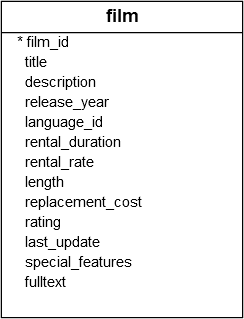### A) A general CASE example

Suppose you want to label the films by their length based on the following logic:

• If the lengh is less than 50 minutes, the film is short.
• If the length is greater than 50 minutes and less than or equal to 120 minutes, the film is medium.
• If the length is greater than 120 minutes, the film is long.

To apply this logic, you can use the `CASE` expression in the `SELECT` statement as follows:

``````SELECT title,
length,
CASE
WHEN length> 0
AND length <= 50 THEN 'Short'
WHEN length > 50
AND length <= 120 THEN 'Medium'
WHEN length> 120 THEN 'Long'
END duration
FROM film
ORDER BY title;
``````

Output: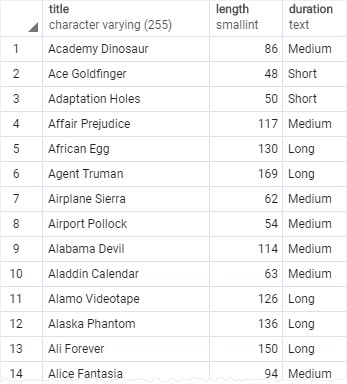Note that we placed a column alias `duration` after the `CASE` expression.

### B) Using CASE with an aggregate function example

Suppose that you want to assign price segments to films with the following logic:

• If the rental rate is 0.99, the film is economic.
• If the rental rate is 1.99, the film is mass.
• If the rental rate is 4.99, the film is premium.

And you want to know the number of films that belong to economy, mass, and premium.

In this case, you can use the `CASE` expression to construct the query as follows:

``````SELECT
SUM (CASE
WHEN rental_rate = 0.99 THEN 1
ELSE 0
END
) AS "Economy",
SUM (
CASE
WHEN rental_rate = 2.99 THEN 1
ELSE 0
END
) AS "Mass",
SUM (
CASE
WHEN rental_rate = 4.99 THEN 1
ELSE 0
END
FROM
film;
``````

The result of the query is as follows: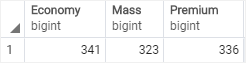In this example, we used the `CASE` expression to return 1 or 0 if the rental rate falls into each price segment. And we applied the `SUM` function to calculate the total of films for each price segment.

## 2) Simple PostgreSQL `CASE` expression

PostgreSQL provides another form of the `CASE` expression called simple form as follows:

``````CASE expression
WHEN value_1 THEN result_1
WHEN value_2 THEN result_2
[WHEN ...]
ELSE
else_result
END
``````

The `CASE` first evaluates the `expression` and compares the result with each value( `value_1`, `value_2`, …) in the `WHEN` clauses sequentially until it finds the match.

Once the result of the `expression` equals a value (value1, value2, etc.) in a `WHEN` clause, the `CASE` returns the corresponding result in the `THEN` clause.

If `CASE` does not find any matches, it returns the `else_result` in that follows the `ELSE`, or `NULL` value if the `ELSE` is not available.

### A) Simple PostgreSQL `CASE` expression example

The following statement uses the `CASE` expression to add the rating description to the output:

``````SELECT title,
rating,
CASE rating
WHEN 'G' THEN 'General Audiences'
WHEN 'PG' THEN 'Parental Guidance Suggested'
WHEN 'PG-13' THEN 'Parents Strongly Cautioned'
WHEN 'R' THEN 'Restricted'
END rating_description
FROM film
ORDER BY title;
``````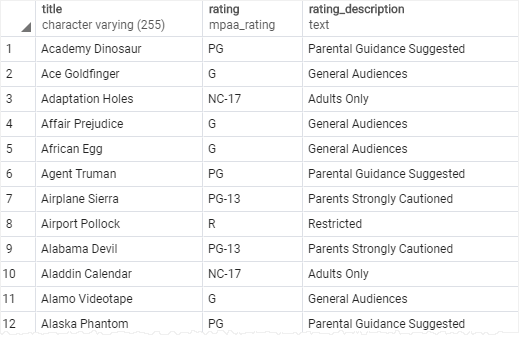In this example, we used a simple `CASE` expression to compare the rating from the `film` table with some literal values like G, PG, NC17, PG-13 and return the corresponding rating description.

### B) Using simple PostgreSQL `CASE` expression with aggregate function example

The following statement uses `CASE` expression with the `SUM` function to calculate the number of films in each rating:

``````SELECT
SUM(CASE rating
WHEN 'G' THEN 1
ELSE 0
END) "General Audiences",
SUM(CASE rating
WHEN 'PG' THEN 1
ELSE 0
END) "Parental Guidance Suggested",
SUM(CASE rating
WHEN 'PG-13' THEN 1
ELSE 0
END) "Parents Strongly Cautioned",
SUM(CASE rating
WHEN 'R' THEN 1
ELSE 0
END) "Restricted",
SUM(CASE rating
WHEN 'NC-17' THEN 1
ELSE 0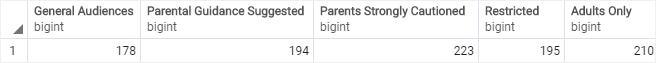In this tutorial, you have learned how to use the PostgreSQL `CASE` expression to form complex queries.